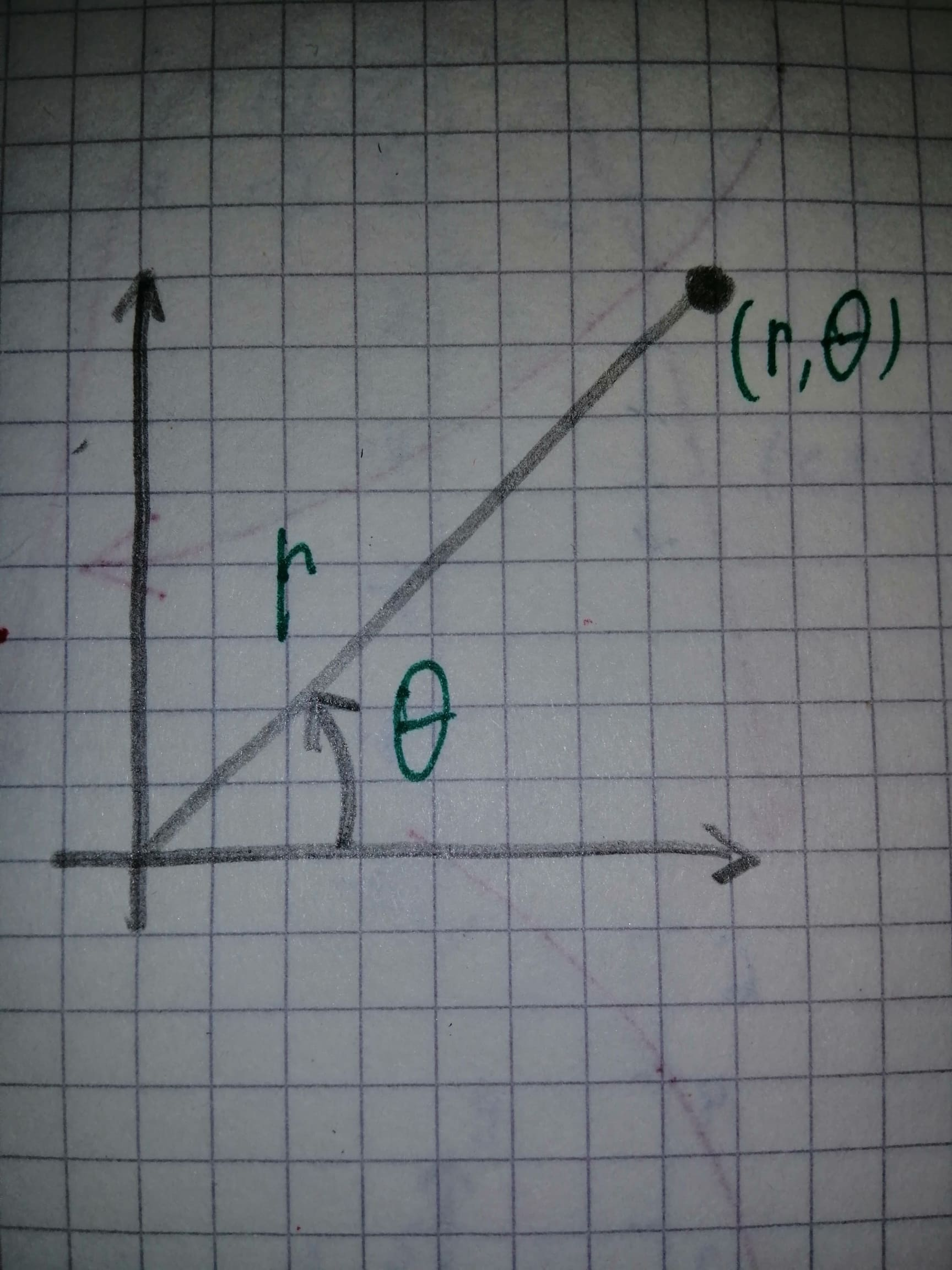# To fill: The blanck spaces in the statement " The origin in the rectangular coordinate system concedes with the ? in polar coordinates. The positive x-axis in rectangular coordinates coincides with the ? in polar coordinates".facas9 2021-02-09 Answered
To fill: The blanck spaces in the statement " The origin in the rectangular coordinate system concedes with the ? in polar coordinates. The positive x-axis in rectangular coordinates coincides with the ? in polar coordinates".
You can still ask an expert for help

• Questions are typically answered in as fast as 30 minutes

Solve your problem for the price of one coffee

• Math expert for every subject
• Pay only if we can solve itensojadasH

The two different ways of locating a point in a plane are the rectangular coordinates and polar coordinates. In polar coordinate system, each point on a plane is determined by a distance from a reference point and an angle from the reference direction. It is represented by $\left(r,\theta \right)$, where r denotes the distance from the reference point and $\theta$ denotes the angle that it makes with the reference direction.
But in the rectangular coordinate system, each point in a plane is determined by a pair of coordinates and represented in the form of (x, y).
So, in rectangular coordinate system the origin coincides with origin in polar and axis in the rectangular coordinate system is the polar axis in the polar coordinate system because an extended line from the reference point is the axis and it denotes the direction of point from the pole in both the coordinate systems.
So, both representations are similar but their nature will vary.
The pole and the polar coordinate arerepresented as the figure given below.